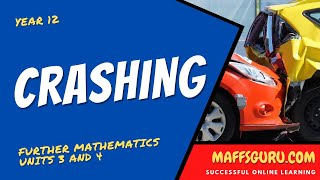Latest Videos
These are the latest videos to be uploaded.
I upload new content each week which covers a range of year levels and topics.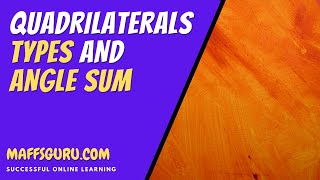Essential Mathematics (Year 8)
Video description:

This video looks at what a quadrilateral is! I know .. you have been doing this for years, but there is new learning to be had. What is a convex and non-convex quadrilateral? What are the different types of quadrilateral? What is the angle sum of a quadrilateral? All these questions (and more!) are answered in this video. There are lots of worked examples all explained in the simplest way.Essential Mathematics (Year 9)
Video description:

This video looks at the amazing properties of parallel lines and a single transversal (a line which passes through two other lines). I look at how the angles which are created can be identified and found using the FUZX rules! Which, in real Maths speak would otherwise be known as, Vertically Opposite Angles, Cointerior Angles, Corresponding Angles and Alternate Angles. Phew! Such long words! There are lots of worked examples all explained in an easy to understand wayEssential Mathematics (Year 8)
Video description:

This video is going to make you hungry as all I talk about it pizza .. and fractions! Starting with the idea of pizza, I look at what equivalent fractions are. I then look at how we can simplify fractions (to get it to the simplest form) and how we can reverse the process to make lots and lots of equivalent fractions. There are a number of worked examples and I make sure to explain the theory in a way you will understand. And, we will ignore the fact that Aussies seem to put an egg on top of one of your pizzas!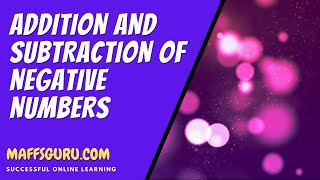Essential Mathematics (Year 8)
Video description:

This video is probably one of the most important for the whole Mathematics curriculum. Negative numbers are used in every subject and topic. Being about to add and subtract negative numbers is going to be vitally important. Sadly, it's something which people find challenging! But ... hopefully not once you've watched this video. Addition and Subtraction of negative numbers can be done using "The Four Rules" which I show (with lots of examples). I also show why they work. All explained without the smoke and mirrors!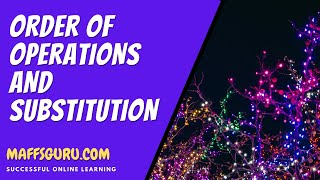Essential Mathematics (Year 8)
Video description:

Before we start, we need to clear up if it's BIDMAS or BODMAS. It's clearly answered in the video and then I take some time to explain how it is used. There are lots of examples which show, not only how to use BIDMAS/BODMAS but how to use it with negative numbers. The rest of the video looks at how we can use the work from Year 7 algebra alongside the new work on negative numbers and BIDMAS. This is the beauty of mathematics! There are lots of worked examples all explained in an easy to understand way.Essential Mathematics (Year 8)
Video description:

This is another really important video which builds on the previous video in this series. Being able to add and subtract negative numbers is really important, but even more important is the ability to multiply and divide them. This comes up in so many parts of the course (Mathematics!). This video looks at a lot of examples and shows how to build on the knowledge from the previous video to answer some pretty complex problems! It's really important to know how to Multiply and Divide IntegersEssential Mathematics (Year 8)
Video description:

Have you ever wondered what the divisibility rules are? Want to know how to find the HCF and LCM of numbers? Do you know that HCF stands for Highest Common Factor and LCM stands for Lowest Common Multiple? Want to know how to do Prime Factorisation using Factor Trees? Then this video will answer all those questions you may - or may not! - have. Lots of worked examples are provided with downloadable notes (at www.maffsguru.com).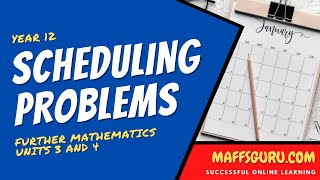Further Mathematics: Units 3 and 4
Video description:

This is the second from last video looking at Networks for the Further Maths course. Scheduling problems is about as real world as it gets! This is practical Mathematics. I take a look at how we can add weights to activity networks to let us schedule tasks e.g. building a house, making a cup of tea etc. I look at forward and backwards scanning. I explain Earliest Start Times (EST) and Latest Start Times (LST) and how they relate to Float Times. We look at how we use the information to find the Minimum completion time and where the Critical Path is. All with lots of examples explained in my own unique way.